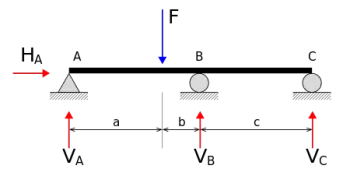# Newton's Third Law: Description of the Law of Action and ReactionNewton's third law (or principle of action and reaction) is the last of the three fundamental laws of dynamics that Sir developed. Isaac Newton together with the law of inertia (1a) and the fundamental law of dynamics (2a). These laws explain much of the aspects related to the movement of bodies.

Newton's third law states that:

"Every action is always opposed by a reaction of equal value, or the mutual actions of two bodies against each other are always directed towards the opposite party."

## Explanation of the Law of Action and Reaction

That is, if a force is exerted on a body a, the body a will respond with another reaction force of equal magnitude and opposite direction.

Newton's first two principles (the law of inertia and the fundamental law of dynamics) refer to a single body. On the other hand, the third law refers to the interaction between two bodies.

Generally, when we talk about the third law we express the F for force with two subscripts: the first of them is to indicate the body that exerts the force, while the second subscript is used to refer to the body on which the force acts.

If, for example, we have two bodies a and b that exert forces between them, Fab represents the force that body “a” exerts on body “b”. In the same way, Fba represents the force that the body "b" exerts on "a": that is, the force exerted by "b" on "a".

Newton's third law states that these two forces are equal and opposite.

The forces occur in pairs, that is, there cannot be a single force applied to an isolated body.

This law is independent of whether the objects are at rest, in motion, or uniform rectilinear motion.

## What Is the Action-reaction Pair?

The action-reaction pair are the two forces that are exerted between two bodies that interact with each other.

Two bodies that exert a force on each other are two objects that interact with each other. Newton's law of action and reaction establishes the relationship between the two forces resulting from the interaction of two bodies. For this reason, the two forces Fab and Fba are sometimes called the action-reaction pair. One of the forces is called an action and the second is called a reaction.

The choice of which force plays the role of action or reaction is an arbitrary decision.

## Examples of Newton's Third Law

• A book that is on a table exerts a force on the table due to gravity. At the same time, the table exerts a normal force with the same magnitude but opposite direction to compensate for this force. Due to this reaction force the book will remain at rest.

• A train machine exerts a force on the wagon attached to it in the forward direction. At the same time, the wagon exerts a reaction force in the opposite direction opposing the advance.

Author:

Published: November 14, 2021
Last review: November 14, 2021